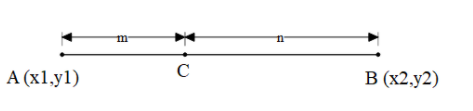QuestionAnswers

# A line perpendicular to the line segment joining the points (1,0) and (2,3) divides it in the ratio 1: n. Find the equation of the line.Hint: In order to solve this problem, we need to understand that the line is divided in a certain ratio and the coordinates of that point are given by section formula. Section formula says that if the segment is divided in ratio m:n between the two coordinates namely $A\left( {{x}_{1}},{{y}_{1}} \right)$ and $B\left( {{x}_{2}},{{y}_{2}} \right)$ then the coordinates that divides are $C\left( \dfrac{m{{x}_{2}}+n{{x}_{1}}}{m+n},\dfrac{m{{y}_{2}}+n{{y}_{1}}}{m+n} \right)$ . We also need to know how to find the slope of the line. The formula for the slope with the same two coordinates $A\left( {{x}_{1}},{{y}_{1}} \right)$ and $B\left( {{x}_{2}},{{y}_{2}} \right)$ is $slope\left( {{m}_{1}} \right)=\dfrac{{{y}_{2}}-{{y}_{1}}}{{{x}_{2}}-{{x}_{1}}}$ .

We are given a with endpoints (1,0) and (2,3) divided in the ratio of 1: n.
We need to find the coordinates of the point that divides the segment.
We can use the section formula for this.Section formula says that if the segment is divided in ratio m:n between the two coordinates namely
$A\left( {{x}_{1}},{{y}_{1}} \right)$ and $B\left( {{x}_{2}},{{y}_{2}} \right)$ then the coordinates that divides are $C\left( \dfrac{m{{x}_{2}}+n{{x}_{1}}}{m+n},\dfrac{m{{y}_{2}}+n{{y}_{1}}}{m+n} \right)$ .
Now for this question let $A\left( {{x}_{1}},{{y}_{1}} \right)=A\left( 1,0 \right)$ and $B\left( {{x}_{2}},{{y}_{2}} \right)=B\left( 2,3 \right)$ .
Applying the formula, we get the coordinates of C as follows,
$C\left( \dfrac{\left( 1\times 2 \right)+\left( n\times 1 \right)}{1+n},\dfrac{\left( n\times 0 \right)+\left( 1\times 3 \right)}{1+n} \right)$
Solving this the coordinates of points C will be,
$C\left( \dfrac{n+2}{1+n},\dfrac{3}{n+1} \right)$
Now we need to find the slope of this line.
The formula for the slope with the same two coordinates $A\left( {{x}_{1}},{{y}_{1}} \right)$ and $B\left( {{x}_{2}},{{y}_{2}} \right)$ is $slope\left( {{m}_{1}} \right)=\dfrac{{{y}_{2}}-{{y}_{1}}}{{{x}_{2}}-{{x}_{1}}}$ .
Substituting the values, we get,
${{m}_{1}}=\dfrac{3-0}{2-1}=3$ .
Now we need to find the slope of the line perpendicular to this line.
But, the product of the slopes of two lines perpendicular to each other is -1.
Hence the slope of other line $\left( {{m}_{2}} \right)$ is as follows,
\begin{align} & {{m}_{1}}.{{m}_{2}}=-1 \\ & 3\times {{m}_{2}}=-1 \\ & {{m}_{2}}=\dfrac{-1}{3}. \\ \end{align}
Now we have to slope and the one point of the line that we have to calculate.
The formula to the equation of the line is as follows,
$\left( y-{{y}_{1}} \right)=m\left( x-{{x}_{1}} \right)$
Substituting $m=\dfrac{-1}{3},{{y}_{1}}=\dfrac{3}{n+1},{{x}_{1}}=\dfrac{n+2}{n+1}$ we get,
$\left( y-\dfrac{3}{n+1} \right)=\dfrac{-1}{3}\left( x-\dfrac{n+2}{n+1} \right)$
Solving this equation, we get,
\begin{align} & 3\left( \dfrac{y\left( n+1 \right)-3}{n+1} \right)=-1\left( \dfrac{x\left( n+1 \right)-\left( n+2 \right)}{n+1} \right) \\ & 3yn+3y-9=-1\left( xn+x-n-2 \right) \\ & \left( 1+n \right)x+3\left( 1+n \right)y=n+11 \\ \end{align}
Therefore, the equation of the line is $\left( 1+n \right)x+3\left( 1+n \right)y=n+11$ .

Note: In this problem, while using the section formula the order is very important as the ratio is not the same, therefore, it is very important of which number multiplies to which number. While calculating the slope can start from any point because as it is the ratio the sign gets neutralized.

View Notes
To Determine the Mass of Two Different Objects Using a Beam BalanceCBSE Class 6 to 12 Maths Formulas - Important Math FormulasCBSE Class 12 Maths Chapter-12 Linear Programming FormulaCBSE Class 10 Maths Chapter 8 - Introduction to Trigonometry FormulaCBSE Class 12 Maths Chapter-7 Integrals FormulaCBSE Class 12 Maths Chapter-4 Determinants FormulaCBSE Class 12 Maths Chapter-3 Matrices FormulaCBSE Class 12 Maths Chapter-13 Probability FormulaTo Find the Weight of a Given Body Using Parallelogram Law of VectorsThe Making of a ScientistImportant Questions for CBSE Class 11 Physics Chapter 3 - Motion in a Straight LineNCERT Books Free Download for Class 11 Physics Chapter 3 - Motion in a Straight LineImportant Questions for CBSE Class 6 English A Pact with The Sun Chapter 9 - What Happened to The ReptilesImportant Questions for CBSE Class 6 English A Pact with The Sun Chapter 8 - A Pact with the SunImportant Questions for CBSE Class 6 English A Pact with The Sun Chapter 6 - The Monkey and the CrocodileImportant Questions for CBSE Class 6 English A Pact with The Sun Chapter 2 - The Friendly MongooseImportant Questions for CBSE Class 6 English A Pact with The Sun Chapter 3 - The Shepherd's TreasureImportant Questions for CBSE Class 6 English A Pact with The Sun Chapter 7 - The Wonder Called SheepImportant Questions for CBSE Class 6 English A Pact with The SunImportant Questions for CBSE Class 6 English A Pact with The Sun Chapter 4 - The Old Clock ShopCBSE Class 12 Maths Question Paper 2020CBSE Class 10 Hindi A Question Paper 2020Hindi A Class 10 CBSE Question Paper 2009Hindi A Class 10 CBSE Question Paper 2015Maths Question Paper for CBSE Class 12 - 2013Hindi A Class 10 CBSE Question Paper 2007Hindi A Class 10 CBSE Question Paper 2013Hindi A Class 10 CBSE Question Paper 2016Hindi A Class 10 CBSE Question Paper 2012Hindi A Class 10 CBSE Question Paper 2010RS Aggarwal Class 6 Solutions Chapter-11 Line Segment, Ray and LineRS Aggarwal Solutions Class 6 Chapter-11 Line Segment, Ray and Line (Ex 11A) Exercise 11.1RS Aggarwal Solutions Class 6 Chapter-11 Line Segment, Ray and Line (Ex 11B) Exercise 11.2RD Sharma Class 12 Maths Solutions Chapter 28 - Straight Line in SpaceClass 11 Physics NCERT solutionsRS Aggarwal Class 12 Solutions Chapter-27 Straight Line in SpaceNCERT Exemplar for Class 11 Physics Chapter 3 - Motion in a Straight Line (Book Solutions)NCERT Solutions for Class 11 Physics Chapter 3 Motion in a Straight Line in HindiRD Sharma Class 12 Solutions Chapter 28 - Straight Line in Space (Ex 28.4) Exercise 28.4RD Sharma Class 12 Solutions Chapter 28 - Straight Line in Space (Ex 28.5) Exercise 28.5